### Create an Account

Home / Questions / For one of the M 48 modules of Example 313 determine a variety of different efficiency va...

# For one of the M 48 modules of Example 313 determine a variety of different efficiency values concerning the conversion of waste heat to electrical energy a Determine the thermodynamic

For one of the M = 48 modules of Example 3.13, determine a variety of different efficiency values concerning the conversion of waste heat to electrical energy.

(a) Determine the thermodynamic efficiency,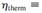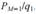(b) Determine the figure of merit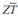for one module, and the thermoelectric efficiency,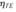using Equation 3.128.

(c) Determine the Carnot efficiency,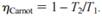(d) Determine both the thermoelectric efficiency and the Carnot efficiency for the case where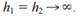(e) The energy conversion efficiency of thermoelectric devices is commonly reported by evaluating Equation 3.128, but with T∞,1 and T∞,2 used instead of T1 and T2, respectively. Determine the value of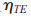based on the inappropriate use of T∞,1 and T∞,2, and compare with your answers for parts (b) and (d).

Jun 28 2020 View more View LessSubscribe To Get Solution